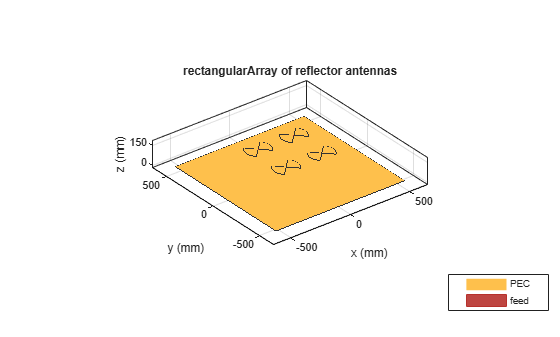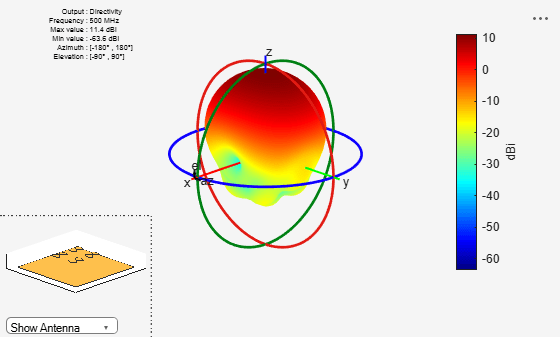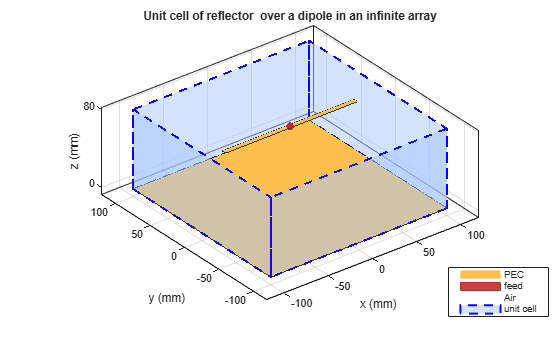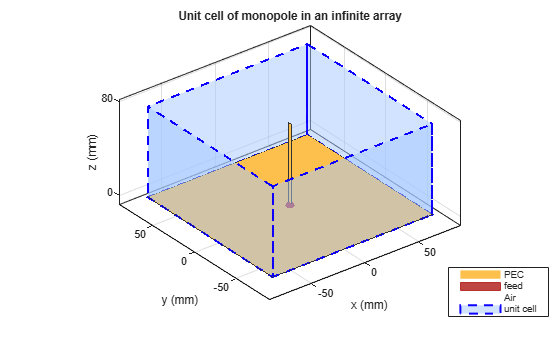Main Content

# design

Design prototype antenna or arrays for resonance at specified frequency

## Syntax

``hant = design(antenna,frequency)``
``harray = design(array,frequency)``
``harray = design(array,frequency,elements)``
``harray = design(conformalarray,frequency)``
``harray = design(conformalarray,frequency,elements)``
``harray = design(infinitearray,frequency)``
``harray = design(infinitearray,frequency,elements)``

## Description

example

````hant = design(antenna,frequency)` designs any antenna object from the antenna library to resonate at the specified frequency.```
````harray = design(array,frequency)` designs an array of dipoles for operation at a specified `frequency`. The elements are separated by half-wavelength.```

example

````harray = design(array,frequency,elements)` designs an array of elements for operation at a specified `frequency`. The elements are separated by half-wavelength, if possible. If you cannot achieve half-wavelength spacing, the element size is used to calculate inter-element separation and the elements are evenly distributed on a sphere radius proportional to the largest element in `element`.```
````harray = design(conformalarray,frequency)` designs a conformal array of dipole and bowtie elements at the specified frequency. The elements are placed in the locations specified by default `conformalArray` object. If the required element positions cannot be achieved due to intersection of elements, the element size is used to compute the inter element spacing and the elements are evenly distributed on a sphere of radius proportional to the largest element in the property `Elements`.```
````harray = design(conformalarray,frequency,elements)` designs a conformal array of specified elements at the specified frequency.```
````harray = design(infinitearray,frequency)` designs an infinite array with a reflector element at the specified frequency.```
````harray = design(infinitearray,frequency,elements)` designs an infinite array of specified elements at the specified frequency.```

## Examples

collapse all

Design a prototype microstrip patch antenna that resonates at a frequency of 1 GHz.

`p = design(patchMicrostrip,1e9)`
```p = patchMicrostrip with properties: Length: 0.1439 Width: 0.1874 Height: 0.0030 Substrate: [1x1 dielectric] GroundPlaneLength: 0.2998 GroundPlaneWidth: 0.2998 PatchCenterOffset: [0 0] FeedOffset: [0.0303 0] Conductor: [1x1 metal] Tilt: 0 TiltAxis: [1 0 0] Load: [1x1 lumpedElement] ```
`show(p)`Calculate the impedance of the above antenna at the same frequency.

`Z = impedance(p,1e9)`
```Z = 55.8475 - 0.8183i ```

Design a rectangular array of reflector backed rounded bowtie antennas to operate at 500 MHz.

```b = bowtieRounded('Tilt',90,'TiltAxis',[0 1 0]); r = reflector('Exciter',b); ra = design(rectangularArray,500e6,r); show(ra)```Plot the radiation pattern of the rectangular array at 500 MHz.

`pattern(ra,500e6)`Create a default conformal array.

`confarraydef = conformalArray`
```confarraydef = conformalArray with properties: Element: {[1x1 dipole] [1x1 bowtieTriangular]} ElementPosition: [2x3 double] Reference: 'feed' AmplitudeTaper: 1 PhaseShift: 0 Tilt: 0 TiltAxis: [1 0 0] ```

Design a conformal array using a dipole antenna, folded dipole antenna, meander dipole antenna, and a monopole antenna at 1 GHz.

`desC = design(confarraydef,1e9,{dipole, dipoleFolded, dipoleMeander, monopole}) `
```desC = conformalArray with properties: Element: {1x4 cell} ElementPosition: [4x3 double] Reference: 'feed' AmplitudeTaper: 1 PhaseShift: 0 Tilt: 0 TiltAxis: [1 0 0] ```
`desC.ElementPosition`
```ans = 4×3 0 0 -1.3016 0 0 -2.6939 0 0 -2.8594 0 0 -3.1498 ```
`show(desC)`Create an infinite array.

`infarrayV1 = infiniteArray`
```infarrayV1 = infiniteArray with properties: Element: [1x1 reflector] ScanAzimuth: 0 ScanElevation: 90 ```
`show(infarrayV1)`Design the above array using a monopole antenna and at 1 GHz frequency.

`infarrayV2 = design(infarrayV1,1e9,monopole)`
```infarrayV2 = infiniteArray with properties: Element: [1x1 monopole] ScanAzimuth: 0 ScanElevation: 90 ```
`show(infarrayV2)`## Input Arguments

collapse all

Antenna object from antenna library, specified as a scalar handle.

Example: `dipole`

Array object from antenna library, specified as a `linearArray`, `rectangularArray`, or `circularArray` object.

Example: ``` r = reflector;ra = design(rectangularArray,500e6,r);``` Designs a rectangular array of reflectors operating at a frequency of 500 MHz.

Conformal array object, specified as a `conformalArray` object.

You can position elements in a conformal array in three ways:

• Case 1: Points lie on a line.

• Case 2: Points lie on a plane.

• Case 3: Points lie in 3-D space.

Example: ```c = conformalArray;ca = design(c,50e6,{dipole,dipoleFolded, dipoleJ, bowtieTriangular,dipole,dipole,dipole,dipole,dipole});``` Designs a conformal array of specified elements operating at a frequency of 50 MHz.

Infinite array object, specified as a `infiniteArray` object.

Example: `i = infiniteArray;ia = design(i,1e9,monopole);` Designs an infinite array with a monopole antenna element operating at a frequency of 1 GHz.

Resonant frequency of the antenna, specified as a real positive scalar.

Example: `55e6`

Data Types: `double`

Antenna object from the antenna library used in the array, specified as a single antenna element or a cell array in conformal array. For more information on element positions for conformal array, see `conformalarray`.

Example: ``` r = reflector;ra = design(rectangularArray,500e6,r); ```Designs a rectangular array of reflectors operating at a frequency of 500 MHz.

Example: ```c = conformalArray;ca = design(c,50e6,{dipole,dipoleFolded, dipoleJ, bowtieTriangular,dipole,dipole,dipole,dipole,dipole});``` Designs a conformal array of specified elements operating at a frequency of 50 MHz.

## Output Arguments

collapse all

Antenna object operating at the specified reference frequency, returned as an antenna object.

Array object operating at the specified reference frequency and specified elements, returned as an array object.

## See Also

Introduced in R2016b

## Support

#### Hybrid Beamforming for Massive MIMO Phased Array Systems

Download the white paper